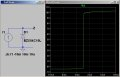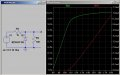# Zener voltage regulator

#### banzai

Joined Jan 3, 2014
71
Hi

I understand that a zener diode will conduct in reverse when it reaches its breakdown voltage, But does it show any resistance to the current flow, lets say a zener breakdown voltage is 10 volts and 16 volts is applied to it in reverse will it act like a variable resistor with respect to the voltage changes (higher voltage lower resistance and vice versa) ?.

How does it do its work in a zener voltage regulator ?thanks in advance guys : )

#### MikeML

Joined Oct 2, 2009
5,444
Dont think of the Zener as a resistor. A resistor it a thing that obeys Ohms law.
Think of it as a device that maintains a more-or-less constant voltage across it even though the current through it is changing.

Look at this simple simulation. I force a current ranging from -10mA to +10mA through a 10V Zener. Notice what the voltage at the cathode of the Zener V(z) plotted along the y-axis does in response to the changing current, which is plotted along the x-axis.Are you surprized what the Zener does for negative currents? How good is this Zener as a voltage regulator for positive currents?

Last edited:
•colinb

#### MikeML

Joined Oct 2, 2009
5,444
Here is sim of a rudimentary Zener regulator, where you are expecting the Zener to keep the voltage across the 10K load resistor R2 constant as the input voltage V1 is changing. I vary V1 from 8V (less than the rated Zener voltage) to 18V along the x-axis. I plot the voltage at node Z V(z) as the green trace left y-axis vs V(v1). Note that this Zener doesn't do anything until V1 reaches about 10.5V. I also plot the current through the Zener -I(D1) as the red trace on the right y-axis.Note that this Zener is not doing real well at keeping the load voltage constant as evidenced by the slope of the green line to the right of the knee. The line would be perfectly horizontal if it were a great regulator...

#### banzai

Joined Jan 3, 2014
71
but technical speaking isn't this done by lets call it dynamic resistance or something like that ?

#### MikeML

Joined Oct 2, 2009
5,444
but technical speaking isn't this done by lets call it dynamic resistance or something like that ?
Yes, and the dynamic impedance can be read directly from the sim I posted in #3, above. Fit a straight line y=mx+b through the V(z) voltages at 2mA and 10mA in the plot. m (the slope) is the dynamic impedance of this Zener

•banzai

#### banzai

Joined Jan 3, 2014
71
t
Yes, and the dynamic impedance can be read directly from the sim I posted in #3, above. Fit a straight line y=mx+b through the V(z) voltages at 2mA and 10mA in the plot. m (the slope) is the dynamic impedance of this Zener
thanks... thats what i wanted to confirm (dynamic resistance), the diode changes its resistance in order to keep the 0.7V voltage drop across it ?

PS

If you dont mind, what is the name of the simulator you used in these pictures ?.

#### MikeML

Joined Oct 2, 2009
5,444
t

thanks... thats what i wanted to confirm (dynamic resistance), the diode changes its resistance in order to keep the 0.7V voltage drop across it ?
No, the Zener diode maintains a constant voltage drop even if the current through it changes. The dynamic impedance of a Zener is a numeric constant (doesn't change) for that particular Zener. Forgot about the Zener changing its resistance; that is a bogus concept, not useful for describing what a Zener does...
If you dont mind, what is the name of the simulator you used in these pictures ?.
LTspiceIV from www.linear.com

Last edited:

#### banzai

Joined Jan 3, 2014
71
tried google but didn't find much. is there a place where one can read about how a diode maintains this voltage drop ?

#### jjw

Joined Dec 24, 2013
501
Hi

I understand that a zener diode will conduct in reverse when it reaches its breakdown voltage, But does it show any resistance to the current flow, lets say a zener breakdown voltage is 10 volts and 16 volts is applied to it in reverse will it act like a variable resistor with respect to the voltage changes (higher voltage lower resistance and vice versa) ?.

How does it do its work in a zener voltage regulator ?thanks in advance guys : )
If you apply 16V to a 10V zener without limiting current it will fry.